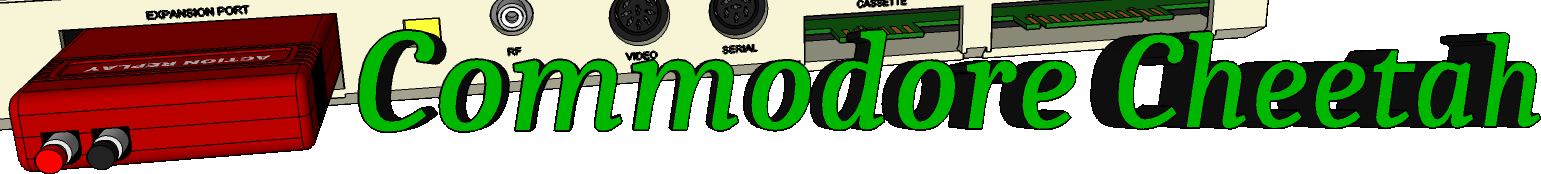Home PageeMail
email5-commodorecheetah.co.uk

Note: You will need to replace the '-' with the @ symbol.
This is to minimize spam.

 My BASIC Quick Reference Guide DIM Abbreviation: D TYPE: Statement FORMAT: DIM ( )[ , ( )...] Action: This statement defines an array or matrix of variables. This allows you to use the variable name with a subscript. The subscript points to the element being used. The lowest element number in an array is zero, and the highest is the number given in the DIM statement, which has a maximum of 32767. The DIM statement must be executed once and only once for each array. A REDIM'D ARRAY error occurs if this line is re-executed. Therefore, most programs perform all DIM operations at the very beginning. There may be any number of dimensions and 255 subscripts in an array, limited only by the amount of RAM memory which is available to hold the variables. The array may be mode up of normal numeric variables, as shown above, or of strings or integer numbers. If the variables are other than normal numeric, use the \$ or % signs after the variable name to indicate string or integer variables, If an array referenced in a program was never DiMensioned, it is automatically dimensioned to 11 elements in each dimension used in the first reference. EXAMPLES of DIM Statement: 10 DIM A(100) 20 DIM Z (5,7), Y(3,4,5) 30 DIM Y7%(Q) 40 DIM PH\$(1000) 50 F(4)=9 : REM AUTOMATICALLY PERFORMS DIM F(10) EXAMPLE of FOOTBALL SCORE-KEEPING Using DIM: 10 DIM S(1,5), T\$(1) 20 INPUT"TEAM NAMES"; T\$(0), T\$(1) 30 FOR Q=1 TO 5: FOR T=0 TO 1 40 PRINT T\$(T),"SCORE IN QUARTER" Q 50 INPUT S(T,Q): S(T,0)= S(T,0)+ S(T,Q) 60 NEXT T,Q 70 PRINT CHR\$(147) "SCOREBOARD" 80 PRINT "QUARTER" 90 FOR Q= 1 TO 5 100 PRINT TAB(Q*2+9) Q; 110 NEXT: PRINT TAB(15) "TOTAL" 120 FOR T=0 TO 1: PRINT T\$(T); 130 FOR Q= 1 TO 5 140 PRINT TAB(Q*2+9) S(T,Q); 150 NEXT: PRINT TAB(15) S(T,0) 160 NEXT CALCULATING MEMORY USED BY DIM: 5 bytes for the array name 2 bytes for each dimension 2 bytes/element for integer variables 5 bytes/element for normal numeric variables 3 bytes/element for string variables 1 byte for each character in each string element

Commodore Cheetah made by Allen Monks, started in the year 2000.